## How to Calculate and Solve for Percentage Yield, Actual Yield and Theoretical Yield in Chemistry | The Calculator Encyclopedia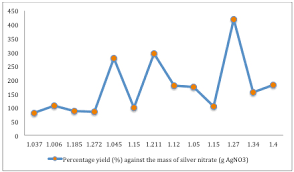The image above represents percentage yield.

To compute the percentage yield, two essential parameters are needed and these parameters are actual yield and theoretical yield.

The formula for calculating percentage yield:

%Yield = (Actual YieldTheoretical Yield) x 100

Let’s solve an example;
Find the percentage yield when the actual yield is 12 with a theoretical yield of 26.

This implies that;
Actual yield = 12
Theoretical yield = 26

%Yield = (Actual YieldTheoretical Yield) x 100
%Yield = (1226) x 100
%Yield = 0.46 x 100
%Yield = 46.15

Therefore, the percentage yield is 46.15.

Calculating the Actual Yield when the Percentage Yield and the Theoretical Yield is Given.

Actual yield = %yield x theoretical yield / 100

Let’s solve an example;
Find the actual yield when the %yield is 52 with a theoretical yield of 10.

This implies that;
%yield = 52
Theoretical yield = 10

Actual yield = %yield x theoretical yield / 100
Actual yield = 52 x 10 / 100
Actual yield = 520 / 100
Actual yield = 5.2

Therefore, the actual yield is 5.2.

## How to Calculate and Solve for the Molar Concentration, Molar Mass and Mass Concentration in Chemistry | The Calculator Encyclopedia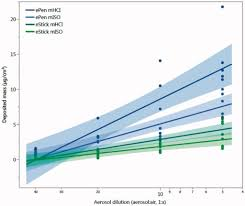The image above represents the mass concentration.

To compute for mass concentration, two essential parameters are needed and these are molar concentration (c) and molar mass (M).

ρ = c x M

Where;
ρ = mass concentration
c = molar concentration
M = molar mass

Let’s solve an example;
Find the mass concentration when the molar concentration is 24 and molar mass is 15.

This implies that;
c = molar concentration = 24
M = molar mass = 15

ρ = c x M
ρ = 24 x 15
ρ = 360

Therefore, the mass concentration is 360 Kg/dm³.

Calculating the Molar Concentration when the Mass concentration and Molar Mass.

c = ρ / M

Where;
c = molar concentration
ρ = mass concentration
M = molar mass

Let’s solve an example;
Find the molar concentration when the mass concentration is 120 with a molar mass of 40.

This implies that;
ρ = mass concentration = 120
M = molar mass = 40

c = ρ / M
c = 120 / 40
c = 3

Therefore, the molar concentration is 3 mol/L.

## How to Calculate and Solve for the Mass, Volume and Mass Concentration in Chemistry | The Calculator Encyclopedia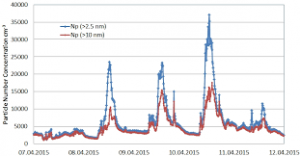The image above represents the mass concentration.

To compute the mass concentration, two essential parameters are needed and these parameters are mass (m) and volume (V).

The formula for calculating mass concentration:

ρ = mV

Where;
ρ = Mass concentration
m = Mass
V = Volume

Let’s solve an example;
Find the mass concentration when the mass is 8 kg with a volume of 24 dm³.

This implies that;
m = Mass = 8
V = Volume = 24

ρ = mV
ρ = 824
ρ = 0.33

Therefore, the mass concentration is 0.33 Kg/dm³.

Calculating the Mass when the Mass Concentration and the Volume is Given.

m = Vρ

Where;
m = Mass
ρ = Mass concentration
V = Volume

Let’s solve an example;
Find the mass when the mass concentration is 12 kg/dm³ with a volume of 7 dm³.

This implies that;
ρ = Mass concentration = 12
V = Volume = 7

m = Vρ
m = 12 x 7
m = 84

Therefore, the mass concentration is 84 kg.

## How to Calculate and Solve for the Number of Moles, Volume and Molar Concentration in Chemistry | Nickzom CalculatorThe image above represents the molar concentration.

To compute the molar concentration of a substance, two essential parameters are needed and these parameters are number of moles (n) and volume (V).

The formula for calculating the molar concentration:

c = nV

Where;
c = Molar Concentration
n = Number of moles
V = Volume

Let’s solve an example;
Find the molar concentration when the number of moles is 32 with a volume of 120.

This implies that;
n = Number of moles = 32
V = Volume = 120

c = nV
c = 32120
c = 0.266

Therefore, the molar concentration is 0.266 mol/L.

Calculating the Number of Moles when Molar Concentration and Volume is Given.

n = Vc

Where;
n = Number of moles
c = Molar Concentration
V = Volume

Let’s solve an example;
Find the number of moles when the molar concentration is 40 with a volume of 31.

This implies that;
c = molar concentration = 40
V = Volume = 31

n = Vc
n = 31 x 40
n = 1240

Therefore, the Number of Moles is 1240 mol.

## How to Calculate and Solve for the Volume of a Constituent, Volume of a Mixture and Volume Concentration in Chemistry | The Calculator EncyclopediaThe image above represents the volume concentration.

To compute the volume concentration of a substance, two essential parameters are needed and these parameters are volume of the constituent (Vi) and volume of the mixture (V).

The formula for calculating volume concentration:

φ = ViV

Where;
φ = Volume concentration
Vi = Volume of the constituent
V = Volume of the mixture

Let’s solve an example;
Find the volume concentration with a volume of the constituent of 23 and a volume of the mixture of 32.

This implies that;
Vi = Volume of the constituent = 23
V = Volume of the mixture = 32

φ = ViV
φ = 2332
φ = 0.718

Therefore, the volume concentration is 0.718.

Calculating the Volume of the Constituent when the Volume Concentration and the Volume of the Mixture is Given.

Vi = Vφ

Where;
φ = Volume concentration
V = Volume of the mixture

Let’s solve an example;
Find the volume of the constituent with a volume concentration of 18 and a volume of the mixture of 22.

This implies that;
φ = Volume concentration = 18
V = Volume of the mixture = 22

Vi = Vφ
Vi = 18 x 22
Vi = 396

Therefore, the volume of the constituent is 396 cm3.

## How to Calculate and Solve for the Number of Entities of a Constituent, Volume and Number Concentration in Chemistry | Nickzom CalculatorThe image above represents the number concentration.

To compute for the number concentration, two essential parameters are needed and these parameters are Number of Entities of the Constituent (N) and Volume (V).

The formula for calculating the number concentration:

C = NV

Where;
C = Number Concentration
N = Number of Entities of the Constituent
V = Volume

Let’s solve an example;
Find the number concentration when the number of entities of the constituent is 12 and the volume is 24.

This implies that;
N = Number of Entities of the Constituent = 12
V = Volume = 24

C = NV
C = 1224
C = 0.5

Therefore, the number concentration is 0.5 m-3.

Calculating the Number of Entities of the Constituent when Number Concentration and Volume is Given.

N = VC

Where;
N = Number of Entities of the Constituent
C = Number Concentration
V = Volume

Let’s solve an example;
Find the number of entities of the constituent when the number concentration is 18 and the volume is 14.

This implies that;
C = Number Concentration = 18
V = Volume = 14

N = VC
N = 14 x 18
N = 252

Therefore, the number of entities of the constituent is 252 m-1.

## How to Calculate and solve for the Mass, Number of Moles and Molar Mass in Chemistry | The Calculator Encyclopedia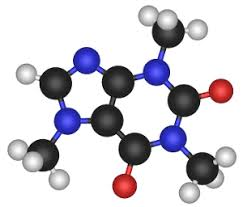The image above represents the molar mass of a substance.

To compute for the molar mass, two essential parameters are needed and these parameters are mass (m) and number of moles (n).

The formula for calculating the molar mass:

M = mn

Where;
M = Molar mass
m = Mass
n = Number of moles

Let’s solve an example;
Find the molar mass of a substance with a mass of 14 kg and number of moles of 28 mol.

This implies that;
m = Mass = 14
n = Number of moles = 28

M = mn
M = 14 ⁄ 28
M = 0.5

Therefore, the molar mass is 0.5 Kg/mol.

Calculating the Mass of a Substance when the Molar Mass and the Number of Moles is Given.

m = nM

Where;
m = Mass
n = Number of moles
M = Molar Mass

Let’s solve an example;
Find the mass of a substance with a molar mass of 30 Kg/mol and number of moles of 15 mol.

This implies that;
n = Number of moles = 15
M = Molar Mass = 30

m = nM
m = 15 x 30
m =  450

Therefore, the mass of a substance is 450 kg.

## How to Calculate and Solve for the number of Particles in a Substance and Number of Moles | The Calculator Encyclopedia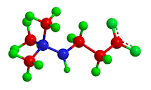The image above represent number of particles in a substance.

To compute for the number of particles in a substance, two essential paramters are needed and these parameters are Avogadro’s constant (h) and number of moles (n).

The formula for calculating the number of particles in a substance:

Number of particles in a substance = n x Avogadro’s constant

Where;
N  = Number of particles in a substance
n = Number of moles

Let’s solve an example:
Find the number of particles in a substance with an Avogadro’s constant of 6.022e+23 and number of moles of 12.

This implies that;
n = Number of moles = 12
h = Avogadro’s constant = 6.022e+23

Number of particles in the substance = 12 x 6.022e+23
Number of particles in the substance = 7.2264e+24

Therefore, the number of particles in the substance is 7.2264e+24 particles.

## Nickzom Calculator Solves Molar Concentration in Chemistry

According to Wikipedia,

Molar concentration (also called molarityamount concentration or substance concentration) is a measure of the concentration of a chemical species, in particular of a solute in a solution, in terms of amount of substance per unit volume of solution. In chemistry, the most commonly used unit for molarity is the number of moles per litre, having the unit symbol mol/L. A solution with a concentration of 1 mol/L is said to be 1 molar, commonly designated as 1 M.

Nickzom Calculator+ (Professional Version) – The Calculator Encyclopedia requires two parameters to get the answer for a molar concentration in Chemistry such as:

• Number of Moles
• Volume

One can access Nickzom Calculator+ (Professional Version) – The Calculator Encyclopedia via any of these channels:

First, go to the calculator map, scroll down to the Chemistry section and click on Basic Chemistry.Now, click on Molar Concentration in Chemistry

## Nickzom Calculator Solves Calculations on Gas Laws

In Physics and Chemistry, there are four prominent gas laws that govern calculations related to gases. These laws have major parameters such as:

• Pressure
• Temperature
• Volume

The four gas laws are:

• Boyle’s Law
• Charles’ Law
• Pressure or Gay Lussac’s Law
• The General Gas Equation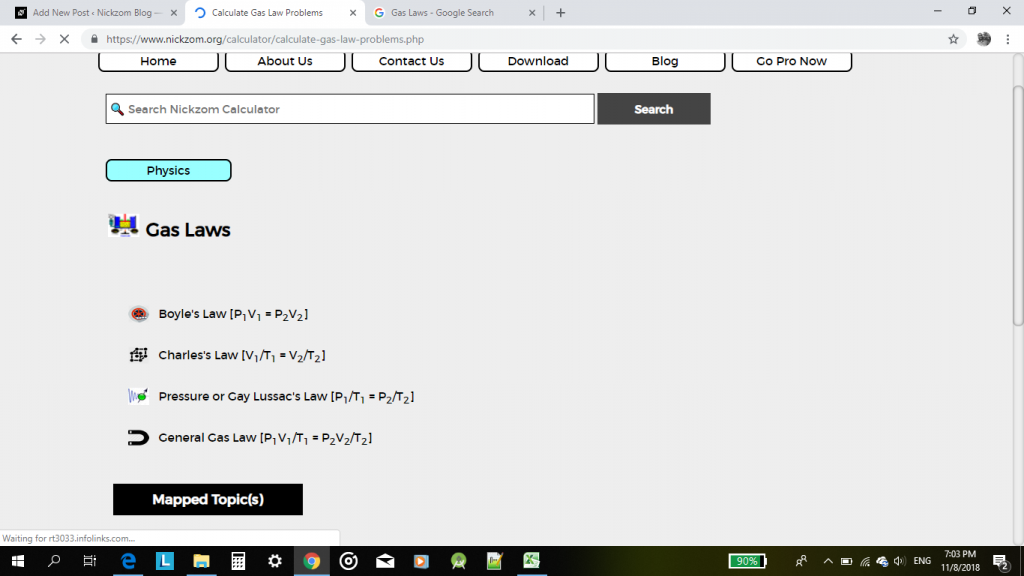According to Boyle’s law, pressure is indirectly proportional to volume.

According to Charles’ law, volume is directly proportional to temperature.

According to Pressure or Gay Lussac’s law, pressure is directly proportional to temperature.

According to the General Gas Equation or law, the product of pressure and volume is directly proportional to temperature.

For this post, I would be given an example of how Nickzom Calculator can solve a problem on gas laws with the general gas equation or law.

One can access Nickzom Calculator via any of these channels:

Let’s assume that one is looking for an unknown temperature.

First and foremost, click on General Gas Law.

Then click on Unknwon Temperature.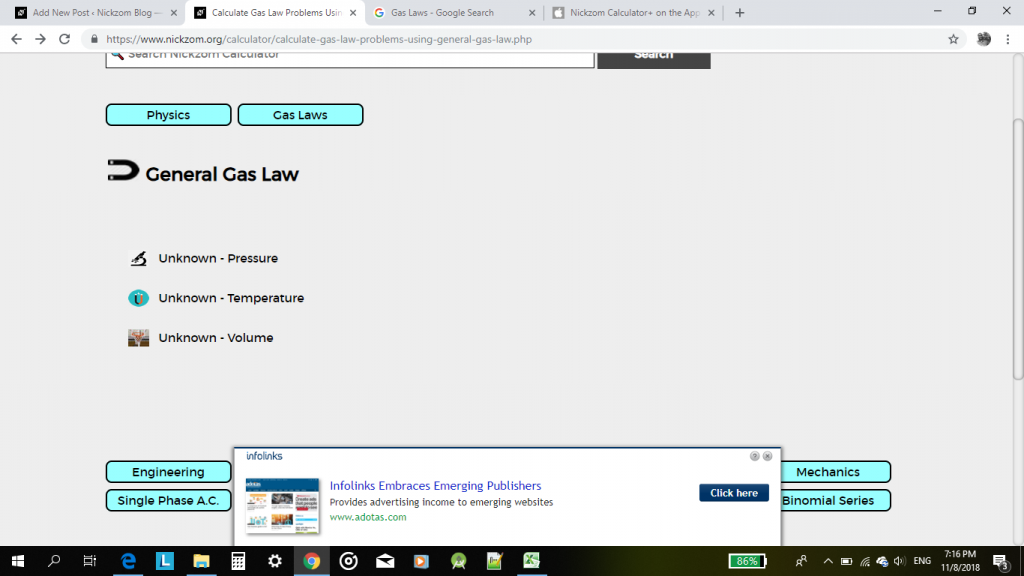Now, proceed to enter the values of the known parameters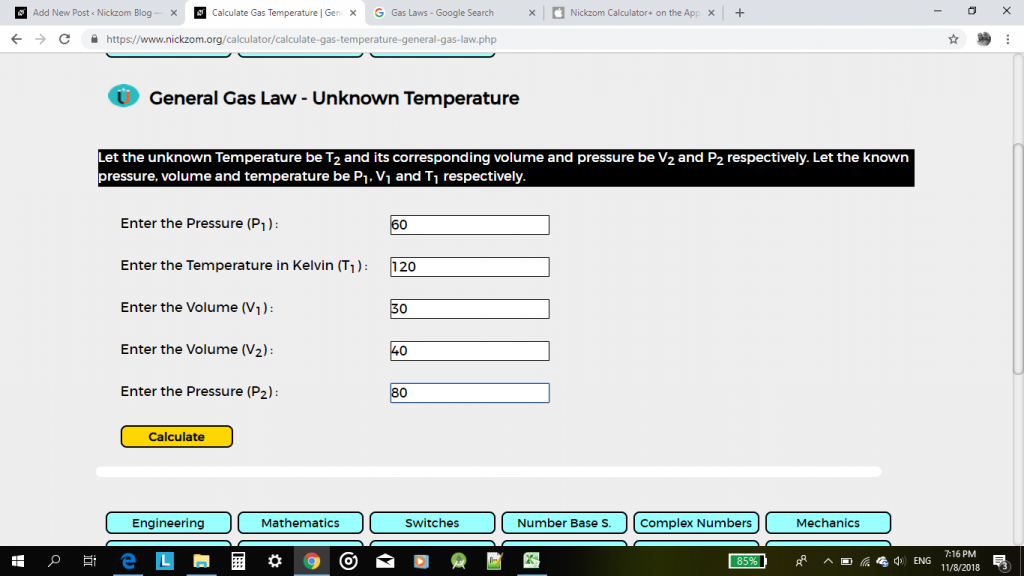Then, click on calculate.

The result would be displayed for you in less than a second.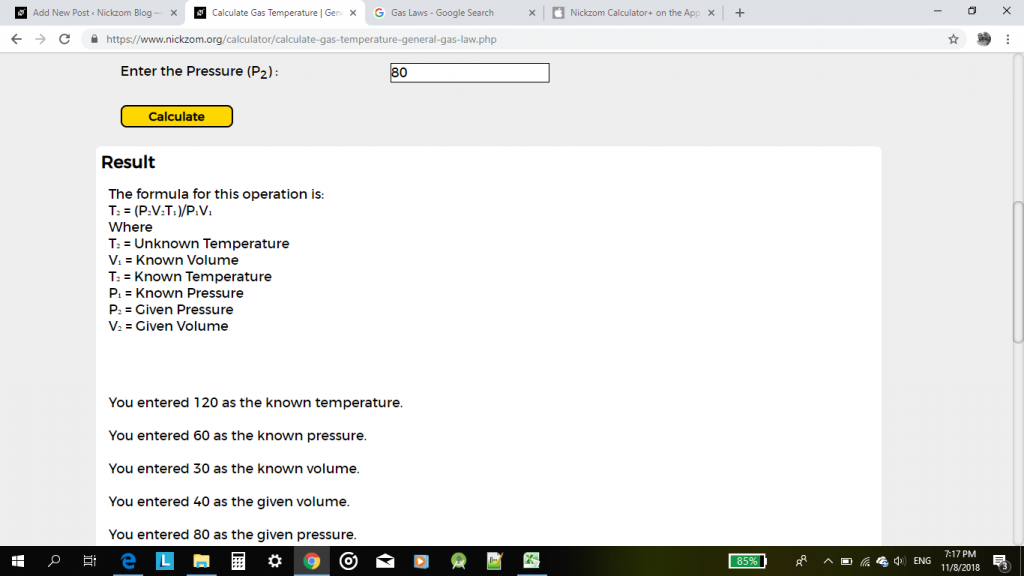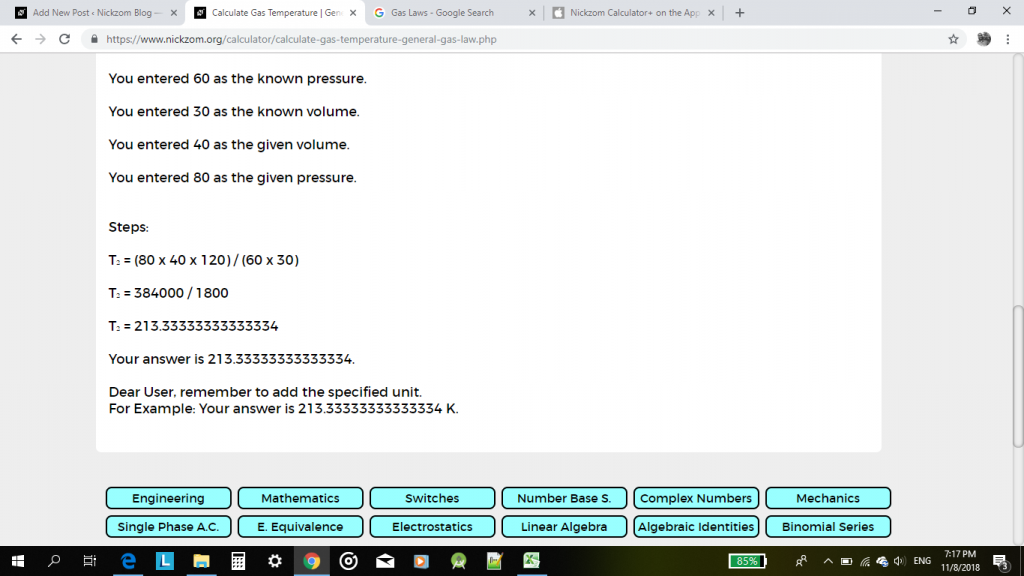This pattern can be used to solve any calculation problem one faces in gas laws.